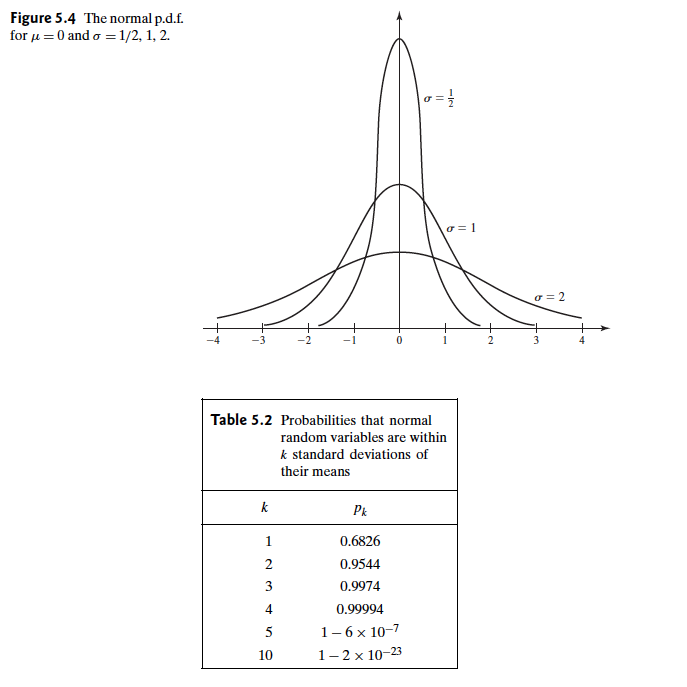Abstract: 本文介绍正态分布第三部分，标准正态分布，正态分布的线性组合，对数正态分布以及对数正态分布
Keywords: The Normal Distributions，The Standard Normal Distribution

# 正态分布

## 标准正态分布 The Standard Normal Distribution

Definition Standard Normal Distribution.The normal distribution with mean 0 and variance 1 is called the standard normal distribution.The p.d.f. of the standard nromal distribution is usually denoted by the symbol $\phi$ ,and the c.d.f. is denoted by the symbol $\Phi$ .Thus,
$$\phi(x)=f(x|0,1)=\frac{1}{(2\pi)^{1/2}}e^{-\frac{1}{2}x^2} \text{ for }-\infty<x<\infty$$
and
$$\Psi(x)=\int^{x}_{-\infty}\Psi(\mu)d\mu \text{ for }-\infty<x<\infty$$

c.d.f是使用初等函数是无法求得的，也就是没有一个封闭的形式，就像本本节开始时说的，只能用查表或者数值法来求p.d.f的某段积分，或者查询c.d.f的结果做差得到对应段的p.d.f.

Theorem Consequences of Symmetry.For all x and all $0 < p < 1$
\begin{aligned} \Psi(-x)=1-\Psi(x) \text{ and } \Psi^{-1}(p)=-\Psi^{-1}(1-p) \end{aligned}

Theorem Converting Normal Distributions to Standard.Let $X$ have the normal distribution with mean $\mu$ and variance $\sigma^2$ .Let $F$ be the c.d.f. of $X$ .Then $Z=(X-\mu)/\sigma$ has the standard normal distribution, and ,for all x and all $0 < p < 1$
$$F(x)=\Phi(\frac{x-\mu}{\sigma})\\ F^{-1}(p)=\mu+\sigma\Phi^{-1}(p)$$

$$Pr(X\leq x)=Pr(Z\leq \frac{x-\mu}{\sigma})$$

Pr(1<X<8)=Pr(\frac{1-5}{2}<\frac{X-5}{2}<\frac{8-5}{2})=Pr(-2<Z<1.5)\\ \text{futhermore:}\\ \begin{aligned} Pr(-1<Z<1.5)&=Pr(Z<1.5)-Pr(Z\leq -2)\\ &=\Phi(1.5)-\Phi(-2)\\ &=\Phi(1.5)-[1-\Phi(2)] \end{aligned}

$$Pr(1<X<8)=0.9105$$

## 正态分布比较 Comparisons of Normal Distributions

$$Pr(\mu_X-k\sigma_X<X<\mu_X+k\sigma_X)=Pr(\mu_Y-k\sigma_Y<Y<\mu_Y+k\sigma_Y)$$

$$p_k=Pr(|X-\mu|\leq k\sigma)=Pr(|Z|\leq k)$$## 正太分布随机变量的线性组合 Linear Combinatios of Normally Distributed Variables

Theorem If the random variables $X_1,\dots,X_k$ are independent and if $X_i$ has the normal distribution with mean $\mu_i$ and variance $\sigma^2_i(i=1,\dots,k)$ ,then the sum $X_1+\dots+X_k$ has the normal distribution with mean $\mu_1+\dots+\mu_k$ and variance $\sigma^2_1+\dots+\sigma^2_k$

$$\Psi(t)=\Pi^{k}_{i=1}\Psi_i(t)=\Pi^k_{i=1}exp[\mu_it+\frac{1}{2}\sigma^2_it^2]\\ =exp{[(\sum^k_{i=1}\mu_i)t+\frac{1}{2}(\sum^{k}_{i=1}\sigma^2_i)t^2]}$$

Corollary If the random variables $X_1,\dots,X_k$ are independent,if $X_i$ has the normal distribution with mean $\mu_i$ and variance $\sigma^2_i (i=1,\dots,k)$ ,and if $a_1,\dots,a_k$ and $b$ are constants for which at least one of the values $a_1,\dots,a_k$ is different from 0,then the variable $a_1X_1+\dots+a_kX_k+b$ has the normal distribution with mean $a_1\mu_1+\dots+a_k\mu_k+b$ and variance $a_1^2\sigma_1^2+\dots+a^2_k\sigma_k^2$

Definition Sample Mean.Let $X_1,\dots,X_n$ be random variables,The average of these $n$ random variables $\frac{1}{n}\sum^{n}_{i=1}X_i$ ,is called their sample mean and is commonly denoted $\bar{X}_n$

Corollary Suppose that the random variables $X_1,\dots,X_n$ form a random sample from the normal distribution with mean $\mu$ and variance $\sigma^2$ ,and let $\bar{X}_n$ denote their sample mean .Then $\bar{X}_n$ has the normal distribution with mean $\mu$ and variance $\sigma^2/n$

$$\bar{X}_n =\sum^{n}_{i=1}(1/n)X_i$$

## 正态分布的对数 The Lognormal Distributions

Definition Lognormal Distribution.If $log(X)$ has the normal distribution with mean $\mu$ and variance $\sigma^2$ ,we say that $X$ has the lognormal distribution with parameters $\mu$ and $\sigma^2$

0%